Win up to 100% scholarship on Aakash BYJU'S JEE/NEET courses with ABNAT Win up to 100% scholarship on Aakash BYJU'S JEE/NEET courses with ABNAT

# JEE Main 2021 March 16 – Shift 1 Chemistry Question Paper with Solutions

JEE Main 2021 March 16th shift 1 Chemistry question paper with solutions are available here. Students can view the questions and also check the correct answers to each of them. The question paper given here will further help students to get familiar with the JEE Main 2021 exam pattern and they will also get to know the marking scheme and the type of questions asked in the entrance exam.

### JEE Main 2021 March 16 – Shift 1 Chemistry Question Paper with Solutions

JEE Main 2021 March 16th Shift 1 Chemistry Question Paper

SECTION-A

Question 1. Among the following, the aromatic compounds are: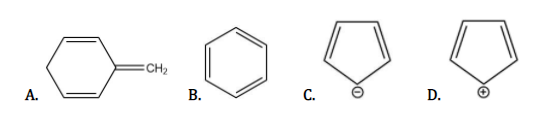Choose the correct answer from the following options:

a. (A) and (B) only
b. (A), (B) and (C) only
c. (B), (C) and (D) only
d. (B) and (C) only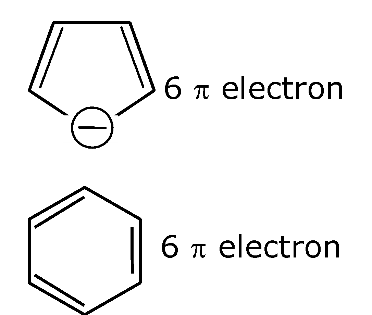Question 2. Given below are two statements:

Statement I: H2O2 can act as both oxidizing and reducing agent in the basic medium.

Statement II: In the hydrogen economy, energy is transmitted in the form of dihydrogen.

In the light of the above statements, choose the correct Ans: from the options given below:

a. Statement I is false but statement II is true
b. Both Statement I and Statement II are true
c. Statement I is true but statement II is false
d. Both Statement I and Statement II are false

H2O2 can act as an oxidizing and reducing agent in both acidic and basic mediums.

Question 3. Which of the following is Lindlar catalyst?

a. Zinc chloride and HCl
c. Sodium and Liquid NH3
d. Cold dilute solution of KMnO4

Lindlar’s catalyst ⇒ Pd/CaCo3 + (CH3COO)2 Pb + quinoline

Question 4. In chromatography technique, the purification of a compound is independent of:

a. Length of the column or TLC plate
b. Mobility or flow of solvent system
c. Physical state of the pure compound
d. Solubility of the compound

Based on NCERT

Question 5. Which among the following pairs of Vitamins is stored in our body relatively for longer duration?

a. Ascorbic acid and Vitamin D
b. Thiamine and Ascorbic acid
c. Vitamin A and Vitamin D
d. Thiamine and Vitamin A

Based on NCERT

Question 6. In the below chemical reaction, intermediate “X” and reagent/condition “A” are: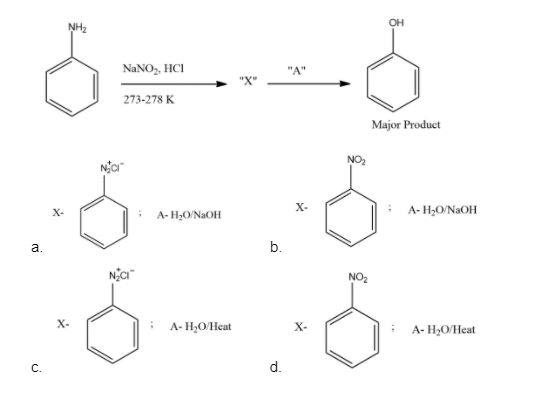Question 7. Which of the following reaction/reactions DOES NOT involve Hoffmann bromamide degradation?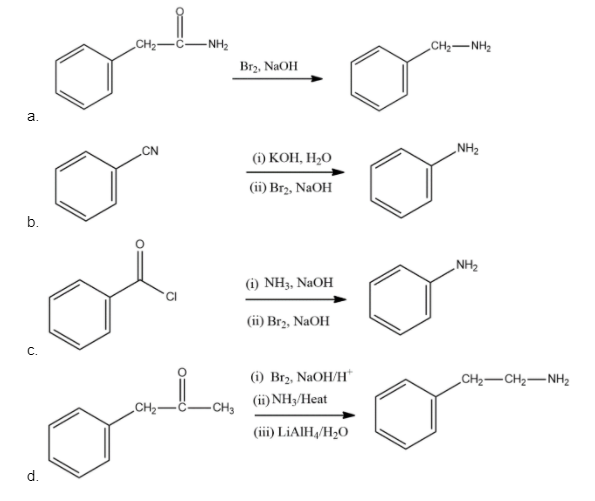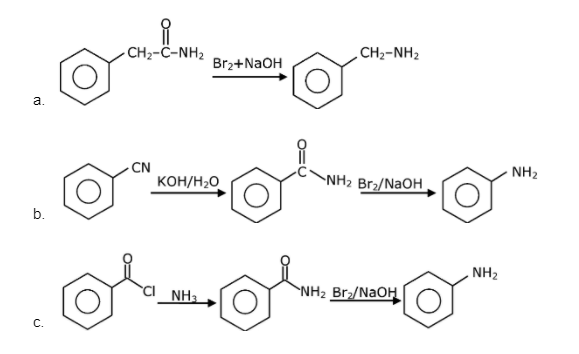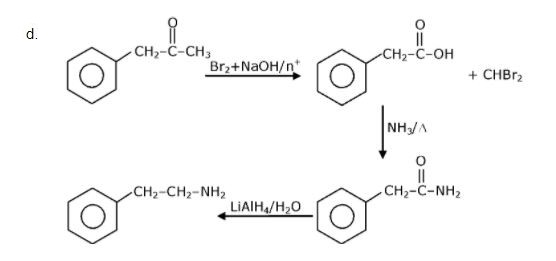Question 8. A group 15 element, which is a metal and forms a hydride with strongest reducing power among group 15 hydrides. The element is:

a. Bi
b. As
c. P
d. S

BiH3 is the strongest reducing agent among the hydrides of 15 group elements as Bi – H bond dissociation energy is very less.

Question 9. Given below are two statements: One is labelled as Assertion A and the other is labelled as Reason R:

Assertion A: The size of the Bk3+ ion is less than the Np3+ ion.

Reason R: The above is a consequence of the lanthanoid contraction.

In the light of the above statements, choose the correct Ans: from the options given below:

a. A is false but R is true
b. Both A and R are true but R is not the correct explanation of A
c. A is true but R is false
d. Both A and R are true and R is the correct explanation of A

93Np3+97Bk3+ as atomic no. increase ionic size decreases. It is due to actinoid contraction.

Question 10. The products “A” and “B” formed in the below reactions are: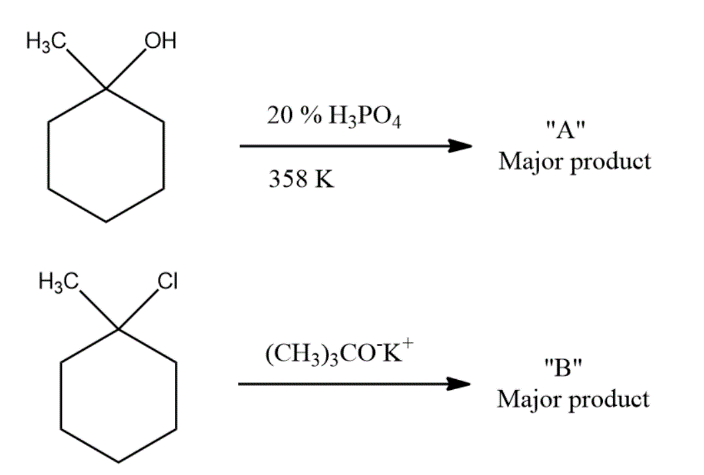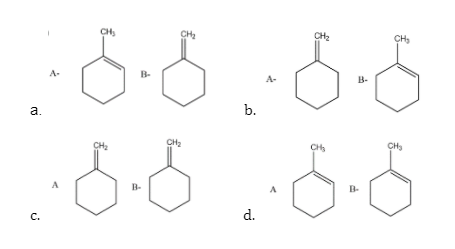Question 11. The type of pollution that gets increased during the day time and in the presence of O3 is:

a. Global warming
b. Reducing smog
c. Acid rain
d. Oxidizing smog

NO + O2 → NO2

NO + O3 → NO2 + O2

$$\begin{array}{l}NO_{2}\overset{hv}{\rightarrow}NO + [O]\end{array}$$

O + O2 → O3

This O3 is called bad ozone.

CH4 + O2 → CH2 = O + H2O

(Vehicle Exhaust)

Question 12. The product “P” in the below reaction is: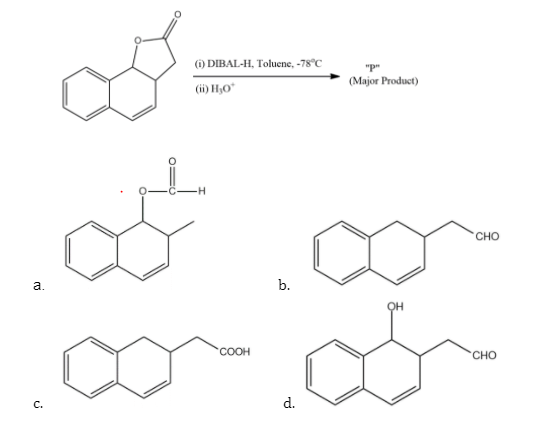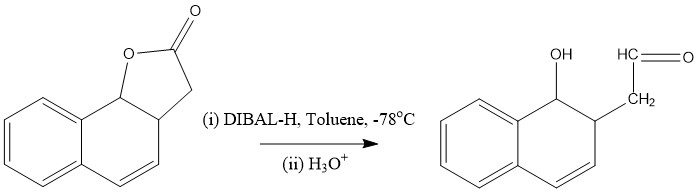Question 13. Match List – I with List – II:

 List-I Industrial process List-II Application (a) Haber’s process (i) HNO3 synthesis (b) Ostwald’s process (ii) Aluminium extraction (c) Contact process (iii) NH3 synthesis (d) Hall-Heroult process (iv) H2SO4 synthesis

Choose the correct Ans: from the options given below:

a. (a)-(iv), (b)-(i), (c)-(ii), (d)-(iii)
b. (a)-(ii), (b)-(iii), (c)-(iv), (d)-(i)
c. (a)-(iii), (b)-(iv), (c)-(i), (d)-(ii)
d. (a)-(iii), (b)-(i), (c)-(iv), (d)-(ii)

Haber’s process is used for NH3 manufacture.

N2 + 3H2 ⇌ 2NH3

Ostwald’s process is used for the preparation of HNO3 by catalytic oxidation of NH3

The contact process is used for the preparation of H2SO4 using V2O5 catalyst

Hall Heroult process is used for Al extraction.

Question 14. Given below are two statements:

Statement I: The Eo value for Ce4+/Ce3+ is +1.74 V.

Statement II: Ce is more stable in Ce4+ state than Ce3+ state.

In the light of the above statements, choose the correct Ans: from the options given below:

a. Both Statement I and Statement II are correct
b. Statement I is incorrect but statement II is correct
c. Both Statement I and Statement II are incorrect
d. Statement I is correct but statement II is incorrect

Ce4+ is a good oxidising agent as Ce3+ is more stable

Ce4- + e → Ce3+

Eo= 1.74 volt

Question 15. Given below are two statements:

Statement I: Both CaCl2.6H2O and MgCl2.8H2O undergo dehydration on heating.

Statement II: BeO is amphoteric whereas the oxides of other elements in the same group are acidic.

In the light of the above statements, choose the correct Ans: from the options given below:

a. Statement I is true but statement II is false
b. Both Statement I and Statement II are false
c. Statement I is false but statement II is true
d. Both Statement I and Statement II are true

$$\begin{array}{l}CaCl_{2}.6H_{2}O\overset{\Delta }{\rightarrow}CaCl_{2} + 6H_{2}O\end{array}$$

MgCl2.6H2O → MgCl(OH) + HCl + 5H2O

Among alkaline earth metal, BeO is amphoteric and the rest are basic oxides.

Question 16. Given below are two statements: One is labelled as Assertion A and the other is labelled as Reason R.

Assertion A: Enol form acetone [CH3COCH3] exists in <0.1% quantity. However, the enol forms acetylacetone [CH3COCH2OCCH3] that exists in approximately 15% quantity.

Reason R: Enol form of acetylacetone is stabilized by intramolecular hydrogen bonding, which is not possible in enol form of acetone.

In the light of the above statements, choose the correct statement:

a. A is true but R is false
b. Both A and R are true but R is the correct explanation of A
c. A is false but R is true
d. Both A and R are true but R is not the correct explanation of A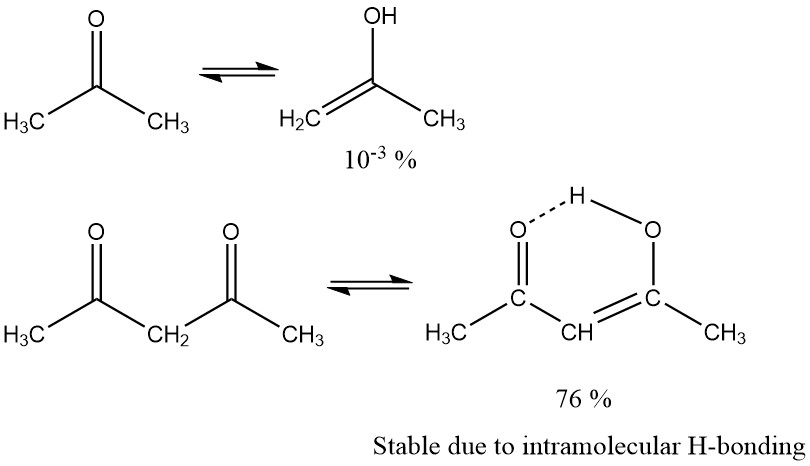Question 17. Given below are two statements: One is labelled as Assertion A and the other is labelled as Reason R

Assertion A: The H–O–H bond angle in a water molecule is 104.5o

Reason R: The lone pair – lone pair repulsion of electrons is higher than the bond pair-bond pair repulsion.

In the light of the above statements, choose the correct Ans: from the options given below:

a. A is false but R is true
b. A is true but R is false
c. Both A and R are true, and R is the correct explanation of A
d. Both A and R are true, but R is not the correct explanation of AIn water O atom is sp3 hybridized with 2 B.P & 2 L.P

Question 18. Match List – I with List – II:

 List-I Name of oxo acid List-II Oxidation state of ‘P’ (a) Hypophosphorous acid (i) +5 (b) Orthophosphoric acid (ii) +4 (c) Hypophosphoric acid (iii) +3 (d) Orthophosphorous acid (iv) +2 – – (v) +1

Choose the correct answer from the options given below:

a. (a)-(iv), (b)-(v), (c)-(ii), (d)-(iii)
b. (a)-(v), (b)-(iv), (c)-(ii), (d)-(iii)
c. (a)-(v), (b)-(i), (c)-(ii), (d)-(iii)
d. (a)-(iv), (b)-(i), (c)-(ii), (d)-(iii)

Hypophosphorous acidH3PO2 +1

Orthophosphoric acid H3PO4 +5

Hypophosphoric acid H4P2O6 +4

Orthophosphoric acid H3PO3 +3

Question 19. The process that involves the removal of sulphur from the ores is:

a. Refining
b. Roasting
c. Smelting
d. Leaching

Roasting removes S as SO2

S + O2 → SO2

Question 20. The functions of antihistamine are:

a. Antiallergic and Analgesic
b. Antacid and antiallergic
c. Antiallergic and antidepressant
d. Analgesic and antacid

Based on NCERT

SECTION-B

Question 21. If the equation below is balanced with integer coefficients, the value of c is__________. (Round off to the nearest integer)

2MnO4 + bC2O2-4 + cH+ → xMn2+ + yCO2 + zH2O.

16H+ + 2MnO4+ 5C2O42–  ⎯→ 2Mn2+ + 10CO2 + 8H2O

Question 22. Complete combustion of 750 g of an organic compound provides 420 g of CO2 and 210 g of H2O. The percentage composition of carbon and hydrogen in organic compounds is 15.3 and _______ respectively. (Round off to the Nearest Integer).

Liebig method:

% of H-element =

$$\begin{array}{l}\frac{2}{18}\times \frac{Mass\: of\: H_{2}O}{Mass\: of\: compound} \times 100\end{array}$$

=

$$\begin{array}{l}\frac{2}{18}\times \frac{210}{750} \times 100 = 3.11\simeq 3\end{array}$$

Question 23. AB2 is 10% dissociated in water to A2+ and B. The boiling point of a 10.0 molal aqueous solution of AB2 is ____________ oC. (Round off to the Nearest Integer).

[Given: Molal elevation constant of water Kb = 0.5 K kg mol–1 boiling point of pure water = 100oC]

ΔTb = iKbm

α = i-1 / n-1

0.1 = i-1 / 3-1 {AB2 ⇌ A2+ + 2B}

i = 1.2

ΔTb = 1.2 × 0.5 × 10 = 6

(Tb)solution = 106°C

Question 24. A certain element crystallizes in a bcc lattice of unit cell edge length 27Å. If the same element under the same conditions crystallises in the fcc lattice, the edge length of the unit cell in Å will be _________. (Round off to the Nearest Integer).

[Assume each lattice point has a single atom]

[Assume √3 = 1.73, √2 = 1.41]

For BCC unit cell, √3a = 4R

a = 4R/√3 = 27

R = 27√3 / 4

For fcc unit cell

√2a = 4R

a = 4/√2 (27√3 / 4)

a = 27 √3 / √2

a = 33.12 ≈ 33

Question 25. The equivalents of ethylene diamine required to replace the neutral ligands from the coordination sphere of the trans-complex of CoCl3.4NH3 is __________. (Round off to the nearest Integer).

COCl3 .4NH3 or [CO(NH3)4Cl2]ClQuestion 26. For the reaction A(g) ⇌ B(g) at 495 K, ΔrGo = –9.478 kJ mol–(1)

If we start the reaction in a closed container at 495 K with 22 millimoles of A, the amount of B in the equilibrium mixture is ________millimoles. (Round off to the nearest Integer).

[R = 8.314] mol–1 K–1; ln 10 = 2.303]

ΔG° = –RT ln Keq

–9.478 × 103 = –495 × 8.314 ln Keq

ln Keq = 2.303 = ln10

So, Keq = 10

Now,

A(g) B(g)

t = 0 22 0

t = t 22–x x

Keq = [B] / [A] = X / (22-X) = 10

x = 20

So, millimoles of B = 20

Question 27. When light of wavelength 248 nm falls on a metal of threshold energy 3.0 eV, the de-Broglie wavelength of emitted electrons is ____________Å. (Round off to the Nearest Integer).

[Use : √3 = 1.73, h = 6.63×10–34 Js

me = 9.1×10-31 kg; c = 3.0×108ms–1; 1eV = 1.6×10–19J]

λ = 248 × 10–9m

w0 = 3 × 1.6 × 10–19 J

E = w0 + K.E.

hc / λ = W0 + K.E.

K.E =

$$\begin{array}{l}K.E = \frac{6.626\times 10\frac{-34}\times 3\times 10^{8}}{248} – 3\times 1.6\times 10^{-19}\end{array}$$

= 3.2 × 10–19J

P = √2mK.E.

P =

$$\begin{array}{l}\sqrt{2\times9.1\times10^{-31}\times3.2\times10^{-19}}\end{array}$$

P = 7.63 × 10–25

$$\begin{array}{l}\lambda = \frac{h}{p}=\frac{6.626\times 10^{-34}}{7.63\times 10^{-25}}\end{array}$$

λ = 8.7 × 10–10 = 8.7Å 9

Question 28. A 6.50 molal solution of KOH (aq.) has a density of 1.89 g cm–3The molarity of the solution is __________ moldm–3 (Round off to the Nearest Integer).

[Atomic masses : K : 39.0 u; O: 16.0 u; H: 1.0 u]

$$\begin{array}{l}m = \frac{1000\times M}{1000\times d-M\times M_{solute}}\end{array}$$
$$\begin{array}{l}6.5 = \frac{1000\times M}{1890\times M\times 56}\end{array}$$

12285 – 364M = 1000M

1364 M = 12285

M = 9

Question 29. Two salts A2X and MX have the same value of solubility product of 4.0×10–12. The ratio of their molar solubilities i.e.

$$\begin{array}{l}\frac{S(A_{2}X)}{S(MX)}\end{array}$$
= _____________. (Round off to the Nearest Integer).

A2X(s) ⇌ 2A+(aq) + X2–(aq)

Solubility: (x) mole / L (2x) (x)

⇒ Ksp = 4 × 10–12 = [A+]2 [X] = 4x3

⇒ x = 10–4 =

$$\begin{array}{l}S_{A_{2}X}\end{array}$$

MX(s) ⇌ M+(aq) + X(aq)

Solubility: (y) mole / L (y) (y)

⇒ Ksp =× 4 10–12 = [M+][X] = y2

⇒ y = 2 × 10–6 = SMX

$$\begin{array}{l}\frac{S(A_{2}X)}{S(MX)} = \frac{10^{-4}}{2\times 10^{-6}} = 50\end{array}$$

Question 30: The decomposition of formic acid on gold surface follows first order kinetics. If the rate constant at 300 K is 1.0×10–3 s–1 and the activation energy Ea = 11.488 kJ mol–1, the rate constant at 200 K is __________×10–5 s–1.(Round off to the Nearest Integer).

$$\begin{array}{l}log\frac{K_{2}}{K_{1}}= \frac{E_{a}}{2.303R}\left [ \frac{1}{T_{1}} = \frac{1}{T_{2}}\right]\end{array}$$
$$\begin{array}{l}log\frac{1.0\times 10^{-3}s^{-1}}{K_{1}}=\frac{11.488\times 1000}{2.303\times 8.314}\left [\frac{1}{200}- \frac{1}{300}\right ]\end{array}$$
$$\begin{array}{l}log\frac{10^{-3}}{K_{1}}=600\times \frac{3-2}{600}\end{array}$$
$$\begin{array}{l}log\frac{10^{-3}}{K_{1}}=1\end{array}$$

$$\begin{array}{l}10 = \frac{10^{-3}}{K_{1}}\end{array}$$

⇒ K1 = 10–4

So, x × 10–5 = 10–4 ⇒ x = 10

(Given: R = 8.314 J Mol–1 K–1)